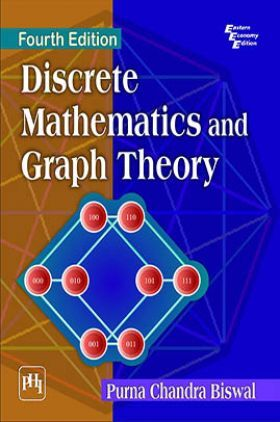# Discrete Mathematics And Graph Theory

Sold ( 53 times )
9225 Views

This product is currently not available.

Save extra with 1 Offers

Get ₹ 50

Instant Cashback on the purchase of ₹ 400 or above

Product Specifications

 Publisher PHI Learning All Engineering Mathematics books by PHI Learning ISBN 9788120350618 Author: BISWAL, PURNA CHANDRA Number of Pages 748 Edition Fourth Edition Available Available in all digital devicesAbout The Book Discrete Mathematics And Graph Theory

Book Summary:

This textbook, now in its fourth edition, continues to provide an accessible introduction to discrete mathematics and graph theory.

The introductory material on Mathematical Logic is followed by extensive coverage of combinatorics, recurrence relation, binary relations, coding theory, distributive lattice, bipartite graphs, trees, algebra, and Polyas counting principle. A number of selected results and methods of discrete mathematics are discussed in a logically coherent fashion from the areas of mathematical logic, set theory, combinatorics, binary relation and function, Boolean lattice, planarity, and group theory. There is an abundance of examples, illustrations and exercises spread throughout the book. A good number of problems in the exercises help students test their knowledge.

The text is intended for the undergraduate students of Computer Science and Engineering as well as to the students of Mathematics and those pursuing courses in the areas of Computer Applications and Information Technology.

New to the Fourth Edition

Introduces new section on Arithmetic Function in Chapter 9.
Elaborates enumeration of spanning trees of wheel graph, fan graph and ladder graph.
Redistributes most of the problems given in exercises section-wise.
Provides many additional definitions, theorems, examples and exercises.
Gives elaborate hints for solving exercise problems.

Preface

Preface to the First Edition

List of Symbols

1. Mathematical Logic

2. Methods of Proof

3. Combinatorics

4. Recurrence Relation

5. Binary Relation and Function

6. Graph Theory

7. Algebraic System and Lattice

8. System with One Binary Operation

9. Finitely Generated Group

10. Homomorphism

11. Counting Principle

12. Permutation Group

13. Sylows Theorem

14. System with Two Binary Operations

15. Coding Theory

Bibliography

Index